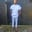Related Tags

php
data
data types
communitycreator

# What are the data types in PHP?Chinweuba Elijah Azubuike

## What are data types?

Data types are various types of data that a variable can store. PHP supports eight data types. In this shot, we'll look at a few of them.

• String: A string is a text written inside quotation marks.

Example

<?php
$text = "Educative"; var_dump($text);

String example

• Integer: Positive and negative whole numbers.

Example

<?php
$number = 12321123; var_dump($number);
Integer example
• Float: Positive and negative numbers with a decimal point.

Example

<?php $float = 123.123; var_dump($float);
Float example
• Boolean: True or false values.

Example

<?php
$x = true;$y = false;
var_dump($x); Boolean example • Array: Collection of similar data. Example <?php$cities = array("Owerri","Telaviv","Washington");
var_dump(\$cities);

Array example

RELATED TAGS

php
data
data types
communitycreator

CONTRIBUTORChinweuba Elijah Azubuike
RELATED COURSES

View all Courses

Keep Exploring

Learn in-demand tech skills in half the time